# Centroid/Volume Detection Chip

### John Dickinson,Scot Myhr, Becky Lund, Jeremy Goldin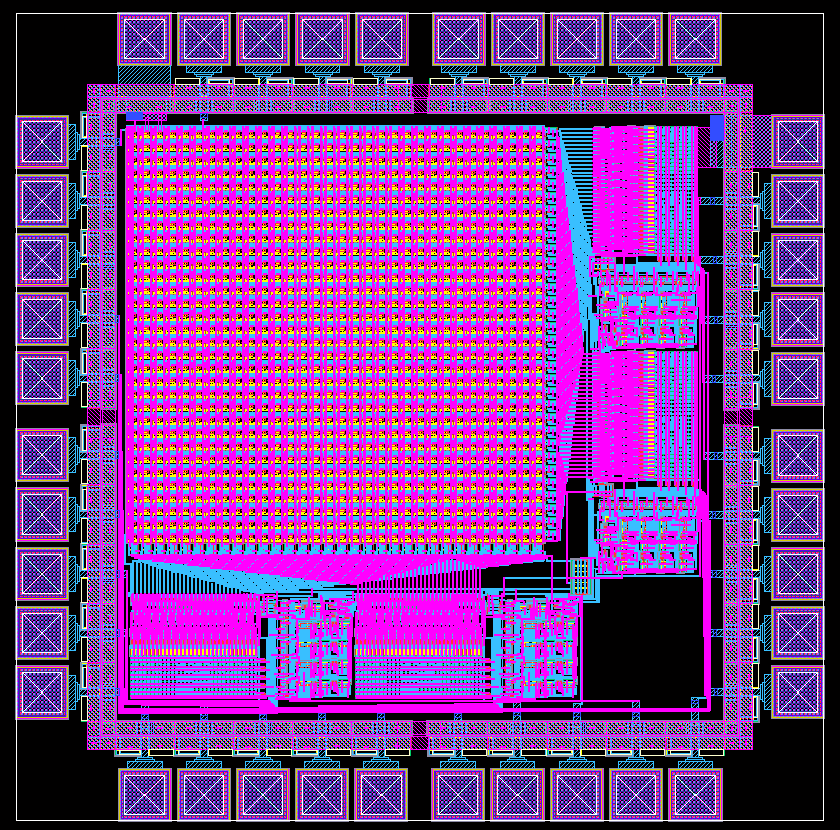Final Schematic

Objectives

The centroid/volume detection imager is designed to detect the centroid of an object and simultaneously give an indication of the volume. One possible application and the motivation behind the design, is the Robocup competition in which robots are built to play soccer and compete with other teams internationally. Since the robots must be autonomous, they need to be able to track objects, such as the ball in a soccer game. By tracking the volume and the center of an object, the robot can tell where and how far away the object is.

Specifications

Our centroid/volume imager contains an array of 32x32 current-mode Active Pixel Sensors (APS) which are capable of detecting light areas and produce a current proportional to the intensity of the bright spot. A current mirror copies the current from each cell to a summation line for each row and column. A switch for each mirror activates the individual rows and columns of the array depending on the location of the position index. Next to the switches we have a 3/4 and 1/4 current divider which produces a percent of the total current summed off of each row and column. We used four current comparators to compare the current percentages with the running sum in order to varry the position index. Four 5-bit counters drive the four address decoders (LUTs) which produces the position index and is utilized to output a parallel coded image position.(BELOW LEFT) Each APS cell outputs two currents that are directly proportional to the light intensity on the photodiode. It uses two bias voltages from off-chip to smooth the signal and control the gain of each cell. One output is sent to the cell's corresponding row and the other to the corresponding column.

(BELOW RIGHT) The current mirrors attach to each row and column. Each mirror makes a copy of the total current coming out of the row/column. The first two copies are sent out to switches, one for the 1/4 comparison and the other for the 3/4 comparison, which turn the row/column on or off, depending on the position index formulated by the majority of volume imaged. For the columns, three copies of the total current from the individual row/columns are made. The extra copy of total current is used on-chip as the total sum and is put off-chip to indicate volume. This third copy need only be produced once and consequently there are only two copies made for the rows.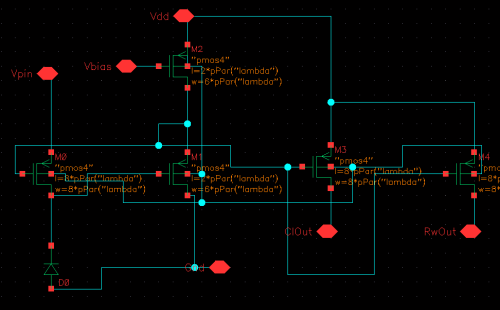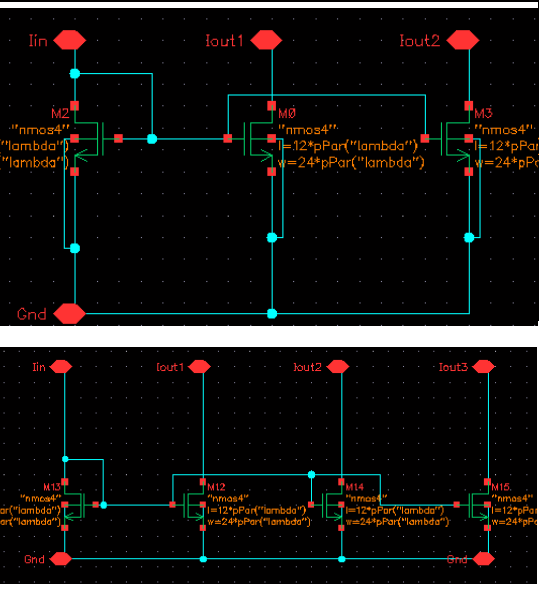(BELOW) There are two switches for each row and each column. These switches allow current from the rows and columns through or not based on the output of the address decoder. The currents that are allowed through are added to make a reference current, I_w. This current is sent to it's respective comparator (1/4 or 3/4). If I_w is greater than 1/4 of the sum, the most sigificant switch is turned off. If it is less than 1/4, the next switch is turned on.(BELOW LEFT) The current divider takes the total current (I_total) copied from the mirrors out of the columns and divides it into 1/4*I_total and 3/4*I_total. It makes two copies of each and sends one pair to the column comparators and the other pair to the row comparators.

(BELOW RIGHT) The comparators take in a current from the current divider and a reference current (I_w) from the switches. It outputs a zero if I_w is less than the I_total fraction to tell the counter to increment. It outputs a one if I_w is greater than the I_total fraction telling the counter to decrement.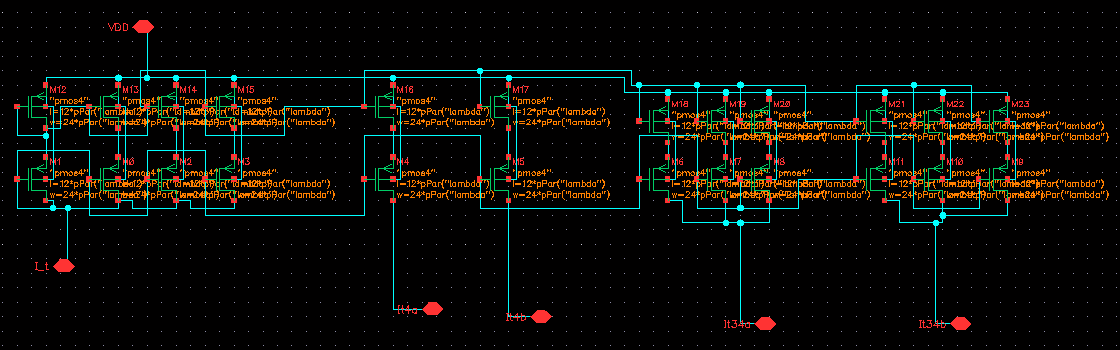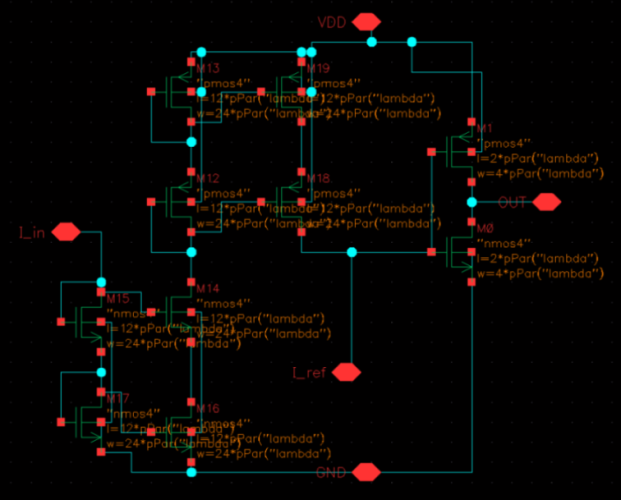(BELOW) The 5-bit counter increments or decrements based on the input from the comparator. Each bit of the counter is sent to an address decoder, indicating the address of where the "pointer" should be. The pointer is defined by the most significant switch that is turned on.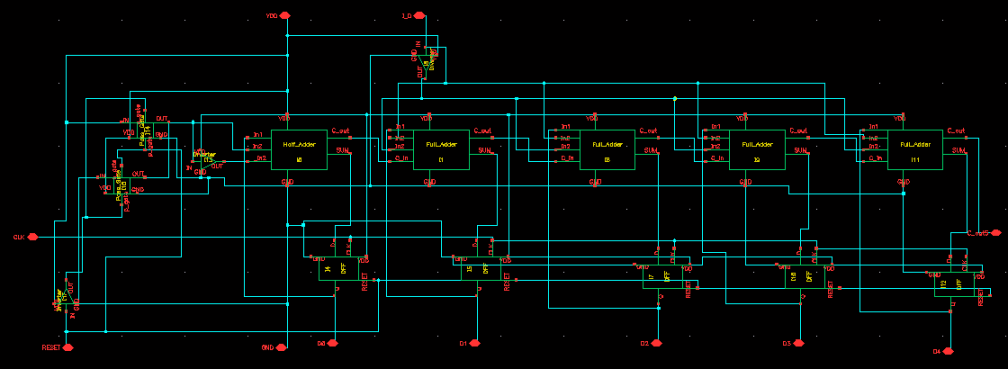The address decoder takes the address outputed from the counter and controls which switches should be on. If the address is all zeros then all the switches would be off, and if the address is all ones, all of the switches would be on. The number of switches open varies linearly with the address.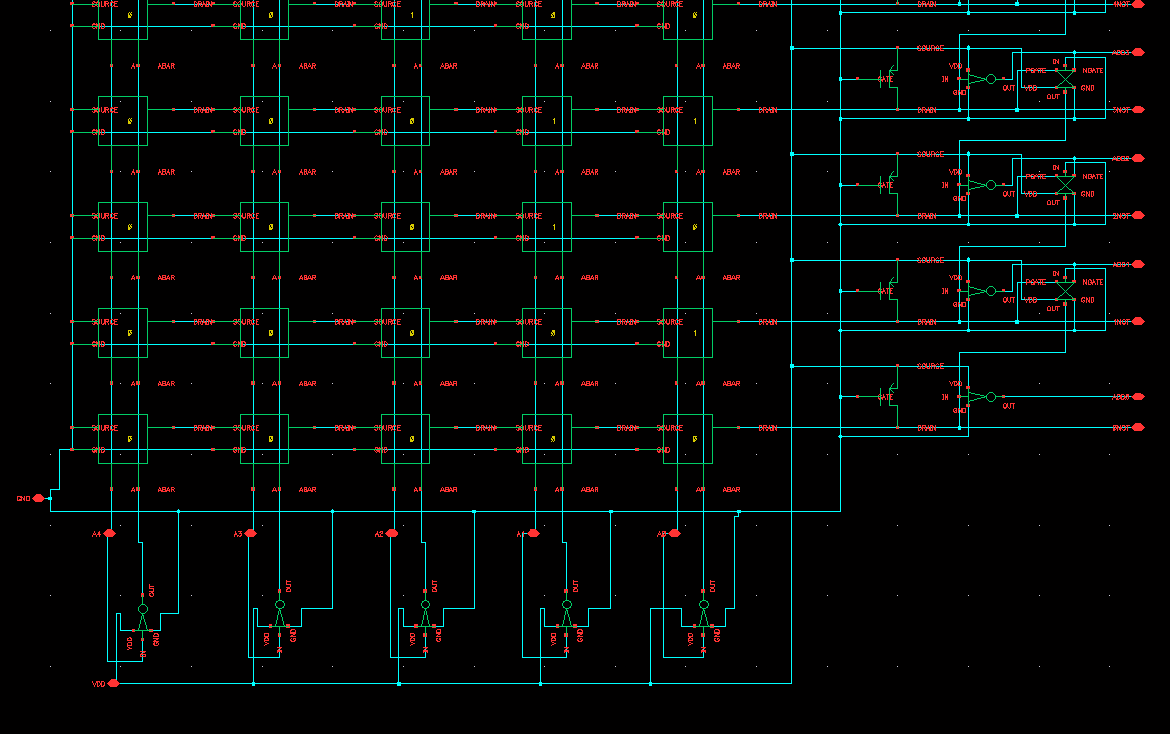Results

This simulation depicts the output of the counter (first five plots) and the comparator (last plot). A hypothetical current distribution was assigned to the columns (or rows) that emulated the current profile for a radial object, such as a small ball for Robocup. The red graph is the least significant bit of the binary counter (and can be seen to switch the most frequently) and the purple is the most significant bit. This plot marks the finding of the 1/4 current position index. The device is activated at the left side of the plot. As the comparisons take place, the address of the position index is increased from 0 (00000) to 11 (01011), at which point it reaches the 1/4 mark and begins to oscilate as the comparator can only indicate an increase or decrease, not equality. Also note that our algorithm is constantly active. As soon as the chip obtains power, it will begin to locate the center of whatever it is pointed at and to output coded locations.## References

Bajic, Ivan V. and John W. Woods, Domain-Based Multiple Description Coding Images and Video. IEEE Transactions on Image Processing, Vol. 12, No. 10, October 2003.

Blum, Richard A., Charles S. Wilson, Paul E. Hasler, and Stephen P. DeWeerth, A CMOS Imager with Real-Time Frame Differencing and Centroid Computation. IEEE 2002.

Clapp, Matthew A. and Ralph Etienne-Cummings, A Dual Pixel-Type Arra for Imaging and Motion Centroid Localization. IEEE Sensors Journal, Vol. 2, No. 6, December 2002.

Cohen, Marc, Gert Cauwenberghs, and Mikhail A. Vorontsov, Image Sharpness and Beam Focus VLSI Sensors for Adaptive Optics. IEEE Sensors Journal, Vol. 2, No. 6, December 2002.

Pui, Hean Boon, Barrie Hayees-Gill, Matt Clark, Mike Somekh, Chung See, Steve Morgan, and Alan Ng, The Design of a Real Time VLSI Optical Centroid Processor. IEEE 2002.